# Equivalence of definitions of pseudocompact space

This article gives a proof/explanation of the equivalence of multiple definitions for the term Pseudocompact space (?)

View a complete list of pages giving proofs of equivalence of definitions

## The definitions that we have to prove are equivalent

1. For any continuous map from the topological space to the real line, the image of the topological space is a closed and bounded subset of the real line.
2. For any continuous map from the topological space to the real line, the image of the topological space is a bounded subset of the real line.
3. Any continuous map from the topological space to the real line attains its absolute maximum and its absolute minimum.

## Proof

We prove that (1) implies both (3), (3) implies (2), and (2) implies (1). The main meat of the proof is in (2) implies (1), because there, we actually need to construct a new function using the original function.

### (1) implies (3)

From (1), the image of a continuous function to the reals is closed and bounded. Since it is bounded, it has a finite supremum and a finite infimum. Since it is closed, the supremum and infimum are both part of the image. Thus, the function attains its absolute maximum and attains its absolute minimum.

### (3) implies (2)

Indeed, this is obvious, because if a function attains an absolute maximum and an absolute minimum, then it must be bounded.

### (2) implies (1)

Given: A topological space$X$ such that for any continuous map from$X$ to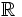$\R$, the image of$X$ is a bounded subset of$\R$. A continuous map$f: X \to \R$.

To prove: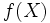$f(X)$ is closed and bounded in$\R$.

Proof: That$f(X)$ is bounded follows directly. We want to prove that$f(X)$ is closed. Suppose not. Then, there exists a real number$a$ that is a limit point of$f(X)$ but not in$f(X)$.

Consider the function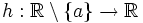$h:\R \setminus \{ a \} \to \R$ given by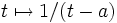$t \mapsto 1/(t - a)$. Set$g = h \circ f$. Then,$g$ is a continuous map from$X$ to$\R$ (it is well-defined because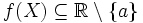$f(X) \subseteq \R \setminus \{ a \}$ and it is continuous because it is a composite of two continuous functions. (Technical point: We are here thinking of$f$ as a map to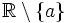$\R \setminus \{ a \}$, and using the fact that a continuous function to another space is also continuous when we reduce the co-domain to a smaller subspace still containing the image of the function).

Thus,$g:X \to \R$ is a continuous function. Moreover, since$f(X)$ contains values arbitrarily close to$a$,$g(X)$ contains values of arbitrarily large magnitude, i.e.,$g(X)$ is unbounded, contradicting our assumption that the image under any continuous function is unbounded.